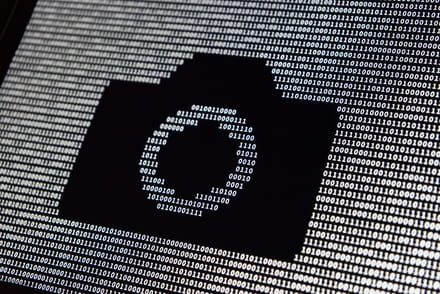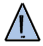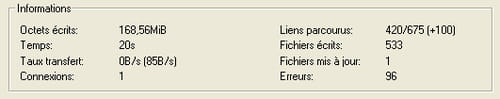# Binary Encoding

In the late 1930s, Claude Shannon showed that by using switches that close for "true" and open for "false," it was possible to carry out logical operations by assigning the number 1 to "true" and 0 for "false." This information encoding system is called binary. It's the form of encoding that allows computers to run. Binary uses two states (represented by the digits 0 and 1) to encode information. This article discusses binary data encoding. You should note that you can find a binary decoder and encoder online.## The Bit

The term bit (shortened to the lowercase b) means binary digit: meaning 0 or 1 in binary numbering. It is the smallest unit of information that can be manipulated by a digital machine. It is possible to represent this binary information: with an electrical or magnetic signal that, beyond a certain threshold, stands for 1; by the roughness of bumps in a surface; and using flip-flops, electrical components that have two stable states (one standing for 1, the other 0).

Therefore, a bit can be set to one of two states: either 1 or 0. With two bits, you can have four different states (2*2):

 0 0 0 1 1 0 1 1

With 3 bits, you can have eight different states (2*2*2):

3-bit binary value Decimal value
000 0
001 1
010 2
011 3
100 4
101 5
110 6
111 7

For a group of n bits, it is possible to represent 2n values.

## Bit Values

In a binary number, a bit's value depends on its position, starting from the right. Like tens, hundreds, and thousands in a decimal number, a bit's value grows by a power of two as it goes from right to left, as shown in the following chart:

 Binary number value 1 1 1 1 1 1 1 1 27 = 128 26 = 64 25 = 32 24 = 16 23 = 8 22 = 4 21 = 2 20 = 1

## Conversion

To convert a binary string into a decimal number, multiply the value of each bit by its weight, then add together the products. Therefore, the binary string 0101, in decimal, becomes:

`23x0 + 22x1 + 21x0 + 20x1= 8x0 + 4x1 + 2x0 + 1x1= 5`

## The Byte

The byte (shortened to the uppercase B) is a unit of information composed of 8 bits. It can be used to store, among other things, a character, such as a letter or number.

Grouping numbers in clusters of 8 makes them easier to read, much as grouping numbers in threes helps to make thousands clearer when working in base-10. For example, the number "1,256,245" is easier to read than "1256245".

A 16-bit unit of information is usually called a word. A 32-bit unit of information is called a double word (sometimes called a dword). For a byte, the smallest number possible is 0 (represented by eight zeroes, 00000000), and the largest is 255 (represented by eight ones, 11111111), making for 256 different possible values.

 27 =128 26 =64 25 =32 24 =16 23 =8 22 =4 21 =2 20 =1 0 0 0 0 0 0 0 0 1 1 1 1 1 1 1 1

## Kilobytes and Megabytes

For a long time, computer science used different values than the metric system for its units. Computer users would often learn that 1 kilobyte was made up of 1024 bytes. For this reason, in December 1998, the International Electrotechnical Commission weighed in on the issue. Here are the IEC's standardized units: one kilobyte (kB) = 1000 bytes ; one megabyte (MB) = 1000 kB = 1,000,000 bytes; one gigabyte (GB) = 1000 MB = 1,000,000,000 bytes; and one terabyte (TB) = 1000 GB = 1,000,000,000,000 bytes.Warning: some software (and even some operating systems) still use the pre-1998 notation, which is as follows: One kilobyte (kB) = 210 bytes = 1024 bytes One megabyte (MB) = 220 bytes = 1024 kB = 1,048,576 bytes One gigabyte (GB) = 230 bytes = 1024 MB = 1,073,741,824 bytes One terabyte (TB) = 240 bytes = 1024 GB = 1,099,511,627,776 bytes

The IEC has also defined binary kilo (kibi), binary mega (mebi), binary giga (gibi), and binary tera (tebi).

They are defined as: one kibibyte (kiB) is worth 210 = 1024 bytes; one mebibyte (MiB) is worth 220 = 1,048,576 bytes; one gibibyte (GiB) is worth 230 = 1,073,741,824 bytes; and one tebibyte (TiB) is worth 240 = 1,099,511,627,776 bytes.

In some languages, such as French and Finnish, the word for byte does not begin with "b", but the international community, on the whole, generally prefers the English term byte. This gives the following notations for kilobyte, megabyte, gigabyte, and terabyte:

`kB, MB, GB, TB`Note the use of an uppercase B to distinguish Byte from bit.

This is a screenshot from the software HTTrack,the most popular offline web browser, showing how this notation is used:## Binary Operations

Simple arithmetic operations — such as addition, subtraction, and multiplication — are easily performed in binary.

Addition in binary follows the same rules as in decimal: start by adding the lowest-valued bits (those on the right) and carry the value over to the next place when the sum of two bits in the same position is greater than the largest value of the unit (in binary: 1). This value is, then, carried over to the bit in the next position.

For example:

 0 1 1 0 1 + 0 1 1 1 0 - - - - - - 1 1 0 1 1

### Multiplication in Binary

The multiplication table in binary is simple:
0x0=0

0x1=0

1x0=0

1x1=1

Multiplication is performed by calculating a partial product for each multiplier (only the non-zero bits will give a non-zero result). When the bit of the multiplier is zero, the partial product is null; when it is equal to one, the partial product is formed from the multiplicand, shifted X places, where X is equal to the weight of the multiplier bit.

For example:

 0 1 0 1 multiplicand x 0 0 1 0 multiplier - - - - - - 0 0 0 0 0 1 0 1 0 0 0 0 - - - - - - 0 1 0 1 0

Image: © Signs and Symbols - Shutterstock.com; Front image: Unsplash